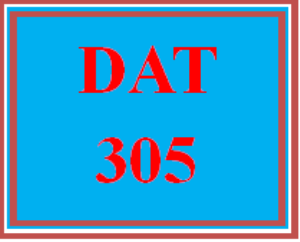# DAT 305 Week 1 Apply - Wk 1 Quiz

PLDZ-12290 In Stock
\$ 8.00 USD
Description

***************************************************************

https://uopcourse.com/category/dat-305/

~~~~~~~~~~~~~~~~~~~~~~~~~~~~~~~~~~~~~~~~~~~~~~~~~

https://uopcourse.com/

***************************************************************

### DAT 305 Week 1 Apply – Wk 1 Quiz

Out of the following list, which runtime complexity scales the worst?

a. O(n2)

b. O(log n)

c. O(1)

d. O(n)

What is the runtime complexity of the following code?

a. O(n)

b. O(1)

c. O(n log n)

d. O(10n)

The binary search algorithm has a big O of which of the following?

a. O(n log n)

b. O(2n)

c. O(log n)

d. O(1)

If we developed an algorithm that performs 5 + 2 log n + n operations, we can say that the algorithm has a complexity of which of the following?

a. O(n)

b. O(5)

c. O(n2)

d. O(log n)

Recent Reviews Write a Review
0 0 0 0 reviews